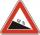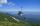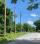The average climb of the road is given by ratio 1:15. By what angle road average climb?

α =  3.81 °

### Step-by-step explanation:Did you find an error or inaccuracy? Feel free to write us. Thank you!Tips to related online calculators
Check out our ratio calculator.

#### You need to know the following knowledge to solve this word math problem:

We encourage you to watch this tutorial video on this math problem:

## Related math problems and questions:

• ClimbRoad has climbing 1:27. How big is a angle corresponds to this climbing?
• ClimbOn the road sign, which informs the climb is 8.7%. The car drive 5 km along this road. What is the height difference that the car went to?On a straight stretch of road is marked 12 percent drop. What angle makes the direction of the road with the horizontal plane?Between cities A and B is route 13 km long of stúpanie average 7‰. Calculate the height difference of cities A and B.
• RailwayRailway line had on 5.8 km segment climb 9 permille. How many meters track ascent?The road sign informs the gradient is 10.9%. Calculate the angle at which the average decreases.
• Traffic signThere is a traffic sign for climbing on the road with an angle of 7%. Calculate at what angle the road rises (falls).The angle of a straight road is approximately 12 degrees. Determine the percentage of this road.
• Height differenceWhat height difference is overcome if we pass a road 1 km long with a pitch21 per mille?
• Map - climbOn the map of High Tatras in scale 1:11000 are cable car stations in the Tatranska Lomnica and in the Skalnate Pleso with distance 354.6 mm. Altitude of this stations are 949 m and 1760 m. What is average angle of climb of this cable car track?
• BevelI have bevel in the ratio 1:6. What is the angle and how do I calculate it?
• StairwayWhat angle rising stairway if step height in 20 cm and width 26 cm?
• Ratio iso triangleThe ratio of the sides of an isosceles triangle is 7:6:7 Find the base angle to the nearest answer correct to 3 significant figure.
• High wallI have a wall 2m high. I need a 15 degree angle (upward) to second wall 4 meters away. How high must the second wall?
• Three pillarsOn a straight road, three pillars are 6 m high at the same distance of 10 m. At what angle of view does Vlado see each pillar if it is 30 m from the first and his eyes are at 1.8 m high?
• PyramidPyramid has a base a = 3cm and height in v = 15 cm. a) calculate angle between plane ABV and base plane b) calculate angle between opposite side edges.
• RiverFrom the observatory 11 m high and 24 m from the riverbank, river width appears in the visual angle φ = 13°. Calculate the width of the river.